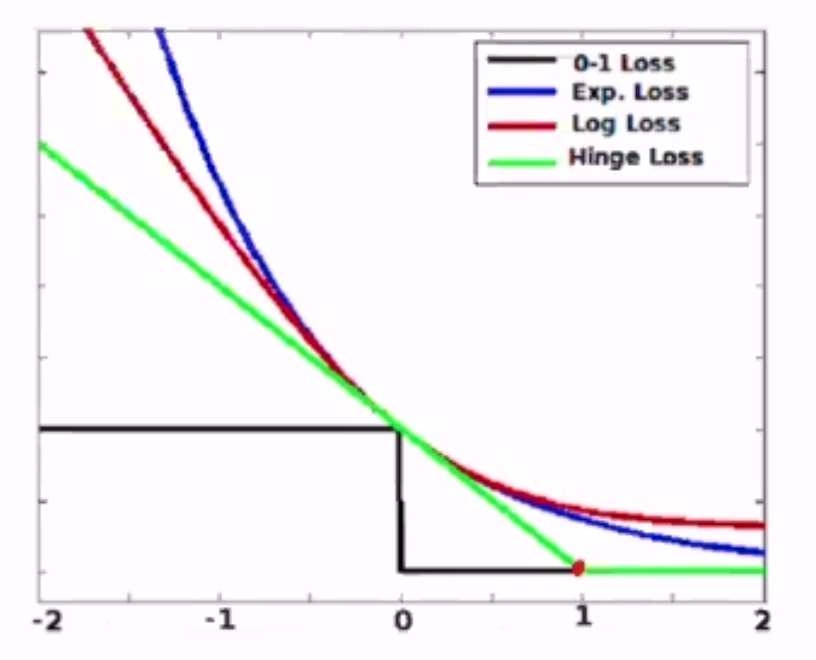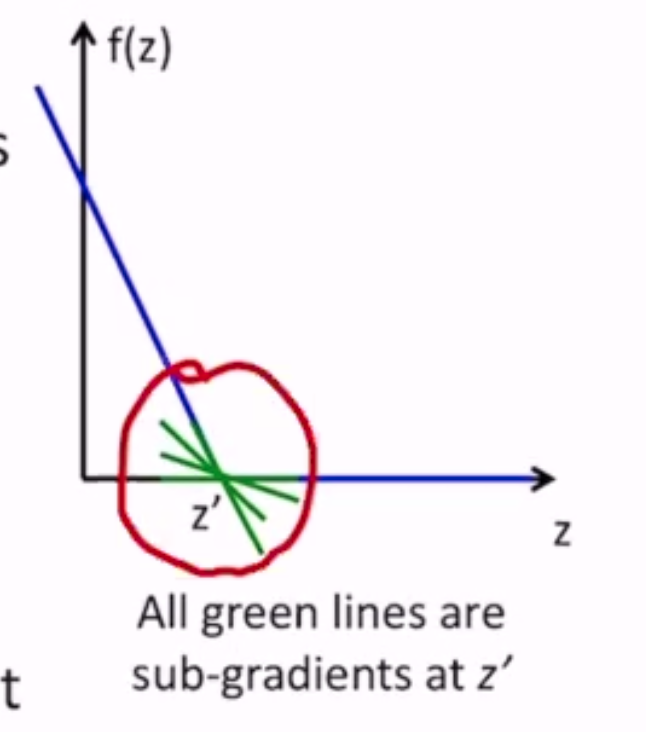# Linear Models¶

If your data is linearly separable, perceptron will find you a separating hyperplane.

But what if my data isn’t linearly separable?

• perceptron will find a hyperplane that makes some errors
• what about a hyperplane that makes a minimal amount of errors?

## Minimum Error Hyperplane¶

The error of a linear model $$(\mathbf{w}, b)$$ for an instance $$(\mathbf{x_n}, y_n)$$ is:

$\mathbf{1} [y_n (\mathbf{w} \cdot \mathbf{x_n} + b) \leq 0]$

where $$\mathbf{1}$$ is an indicator function that returns 1 on incorrect prediction and 0 on correct (0-1 loss)

Based on this, we can make an objective to minimize the minimum error hyperplane:

$\min_{\mathbf{w}, b} \sum_n \mathbf{1} [y_n (\mathbf{w} \cdot \mathbf{x_n} + b) \leq 0]$

This is ERM: empirical risk minimization.

But there are problems:

• the loss fcn is not convex
• not differentiable

### Alternatives to 0-1 Loss¶

We need to find an upper-bound to 0-1 loss that is convex, so that minimization is easy. Also, minimizing the upper bound of the objective pushes down the real objective.

Given $$y, a$$ (label, activation):

• 0/1: $$l^{(0/1)}(y, a) = 1[ya \leq 0]$$
• hinge: $$l^{(hin)}(y, a) = \max\{0, 1-ya\}$$
• logistic: $$l^{(log)}(y, a) = \frac{1}{\log 2} \log(1 + \exp[-ya])$$
• exponential: $$l^{(exp)}(y, a) = \exp[-ya]$$These are all convex functions and can be minimized using SGD - except for hinge loss at point 1.

• sub-gradient of $$f(z)$$ at a point $$z'$$ is the set of all lines that touch $$f(z)$$ at $$z'$$ but lie below $$f(z)$$